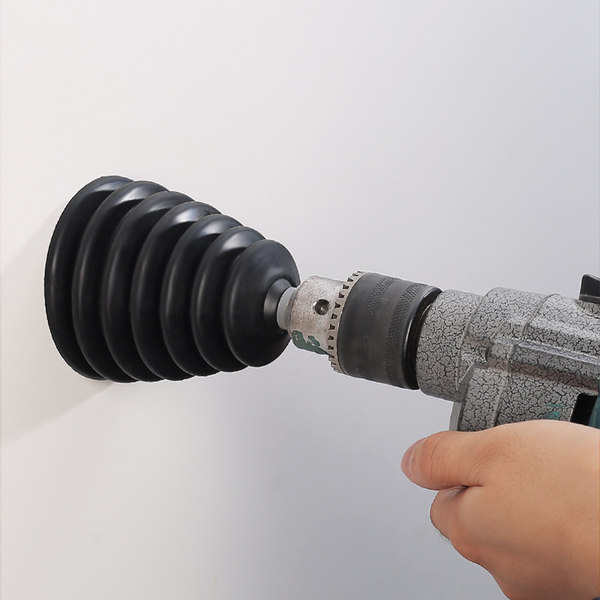﻿ 加减法口诀表(加减法口诀表) - 冯晓强博客

加减法口诀表(加减法口诀表)加减法口诀表

10以内加法口诀

1+ 1= 2

1+ 2= 3 2+ 2= 4

1+ 3= 4 2+ 3= 5 3+ 3= 6

1+ 4= 5 2+ 4= 6 3+ 4= 7 4+ 4= 8

1+ 5= 6 2+ 5= 7 3+ 5= 8 4+ 5= 9 5+ 5=10

1+ 6= 7 2+ 6= 8 3+ 6= 9 4+ 6=10 5+ 6=11 6+ 6=12

1+ 7= 8 2+ 7= 9 3+ 7=10 4+ 7=11 5+ 7=12 6+ 7=13 7+ 7=14

1+ 8= 9 2+ 8=10 3+ 8=11 4+ 8=12 5+ 8=13 6+ 8=14 7+ 8=15 8+ 8=16

1+ 9=10 2+ 9=11 3+ 9=12 4+ 9=13 5+ 9=14 6+ 9=15 7+ 9=16 8+ 9=17 9+ 9=18

1+10=11 2+10=12 3+10=13 4+10=14 5+10=15 6+10=16 7+10=17 8+10=18 9+10=19

10+10=20

20以内加法口诀

1+11=12 2+11=13 3+11=14 4+11=15 5+11=16 6+11=17 7+11=18 8+11=19 9+11=20

1+12=13 2+12=14 3+12=15 4+12=16 5+12=17 6+12=18 7+12=19 8+12=20

1+13=14 2+13=15 3+13=16 4+13=17 5+13=18 6+13=19 7+13=20

1+14=15 2+14=16 3+14=17 4+14=18 5+14=19 6+14=20

1+15=16 2+15=17 3+15=18 4+15=19 5+15=20

1+16=17 2+16=18 3+16=19 4+16=20

1+17=18 2+17=19 3+17=20

1+18=19 2+18=20

1+19=20

10以内减法口诀

9-9=0 9-8=1 9-7=2 9-6=3 9-5=4 9-4=5 9-3=6 9-2=7 9-1=8

8-8=0 8-7=1 8-6=2 8-5=3 8-4=4 8-3=5 8-2=6 8-1=7

7-7=0 7-6=1 7-5=2 7-4=3 7-3=4 7-2=5 7-1=6

6-6=0 6-5=1 6-4=2 6-3=3 6-2=4 6-1=5

5-5=0 5-4=1 5-3=2 5-2=1 5-1=4

4-4=0 4-3=1 4-2=2 4-1=3

3-3=0 3-2=1 3-1=2

2-2=0 2-1=1

1-1=0

20以类加减法口诀表

10以内加减法的口算口诀

10以内加法口诀

1+1=2 1+2=3 1+3=4 1+4=5 1+5=6 1+6=7 1+7=8 1+8=9 1+9=10

2+1=3 2+2=4 2+3=5 2+4=6 2+5=7 2+6=8 2+7=9 2+8=10 2+9=11

3+1=4 3+2=5 3+3=6 3+4=7 3+5=8 3+6=9 3+7=10 3+8=11 3+9=12

4+1=5 4+2=6 4+3=7 4+4=8 4+5=9 4+6=10 4+7=11 4+8=12 4+9=13

5+1=6 5+2=7 5+3=8 5+4=9 5+5=10 5+6=11 5+7=12 5+8=13 5+9=14

6+1=7 6+2=8 6+3=9 6+4=10 6+5=11 6+6=12 6+7=13 6+8=14 6+9=15

7+1=8 7+2=9 7+3=10 7+4=11 7+5=12 7+6=13 7+7=14 7+8=15 7+9=16

8+1=9 8+2=10 8+3=11 8+3=11 8+4=12 8+5=13 8+6=14 8+7=15 8+8=16

9+1=10 9+2=11 9+3=12 9+4=13 9+5=14 9+6=15 9+7=16 9+8=17 9+9=18

10以内减法口诀

9-9=0 9-8=1 9-7=2 9-6=3 9-5=4 9-4=5 9-3=6 9-2=7 9-1=8

8-8=0 8-7=1 8-6=2 8-5=3 8-4=4 8-3=5 8-2=6 8-1=7

7-7=0 7-6=1 7-5=2 7-4=3 7-3=4 7-2=5 7-1=6

6-6=0 6-5=1 6-4=2 6-3=3 6-2=4 6-1=5

5-5=0 5-4=1 5-3=2 5-2=1 5-1=4

4-4=0 4-3=1 4-2=2 4-1=3

3-3=0 3-2=1 3-1=2

2-2=0 2-1=1

1-1=0 20以内加减混合计算

1) 9+1-9= 2) 8+4-8= 3) 5+9-8= 4) 9+9-8= 5) 8+3-8=

6) 6+5-6= 7) 9+1-5= 8) 10+8-7= 9) 7+9-2= 10) 7+8-6=

11) 4+8-4= 12) 8-4+8= 13) 7+9-9= 14) 7-2+9= 15) 3+7-6=

16) 7+5-3= 17) 10-6+6= 18) 7+3-4= 19) 2+10-1= 20) 4+7-9=

21) 9+10-7= 22) 7+10-1= 23) 7+5-8= 24) 5+10-7= 25) 9+7-1=

26) 9+2-1= 27) 10-3+5= 28) 7+8-9= 29) 8-2+6= 30) 10-4+4=

31) 9-1+10= 32) 4+7-2= 33) 5+6-5= 34) 8+6-10= 35) 8-5+10=

36) 9-3+4= 37) 3+9-4= 38) 6+7-7= 39) 5+6-1= 40) 10+10-9=

41) 2+9-7= 42) 7+10-8= 43) 6-1+9= 44) 4+10-9= 45) 2+10-4=

46) 8-1+8= 47) 8+6-4= 48) 10+1-3= 49) 10+2-2= 50) 9+9-5=

51) 10+7-8= 52) 8+2-9= 53) 6+8-3= 54) 8+3-3= 55) 1+9-4=

56) 6+5-9= 57) 6+4-6= 58) 9+4-3= 59) 9+10-4= 60) 10+4-10=

61) 9+1-2= 62) 10-8+8= 63) 6+8-7= 64) 4+9-4= 65) 7-5+9=

66) 8+9-1= 67) 9+2-10= 68) 9-7+8= 69) 8+8-2= 70) 9+5-8=

71) 7-4+9= 72) 2+8-8= 73) 8+4-5= 74) 10+6-8= 75) 8+5-7=

76) 5+6-10= 77) 10+8-1= 78) 4+8-10= 79) 4+6-10= 80) 10+3-5=

81) 6+9-8= 82) 10+1-7= 83) 7+7-3= 84) 8+5-1= 85) 10-1+4=

86) 8+5-5= 87) 10+4-1= 88) 9+3-10= 89) 9-2+5= 90) 7-6+10=

91) 6+7-10= 92) 10+7-2= 93) 5+5-8= 94) 1+10-7= 95) 10-1+7=

96) 5+5-5= 97) 8+9-7= 98) 5-3+9= 99) 5+7-6= 100) 10-2+8=

20以内连加、连减

1) 6+6+1= 2) 4+9+1= 3) 20-8-6= 4) 8+10+1= 5) 4+6+9=

6) 1+4+3= 7) 6+5+3= 8) 8-6-1= 9) 3+8+7= 10) 3+7+5=

11) 19-3-2= 12) 13-10-2= 13) 3+10+4= 14) 12-7-2= 15) 17-10-5=

16) 2+10+4= 17) 16-4-7= 18) 2+6+5= 19) 17-7-1= 20) 19-2-1=

21) 9+2+8= 22) 4+1+2= 23) 3+1+8= 24) 20-10-5= 25) 8+1+6=

26) 6+6+6= 27) 15-10-4= 28) 3+8+2= 29) 13-5-6= 30) 16-2-1=

31) 14-5-2= 32) 18-10-4= 33) 20-1-7= 34) 5+4+3= 35) 14-2-1=

36) 14-7-4= 37) 18-4-6= 38) 1+8+8= 39) 19-10-8= 40) 10-1-7=

41) 17-6-4= 42) 4+2+6= 43) 11-8-1= 44) 19-8-2= 45) 17-7-9=

46) 13-4-3= 47) 5+3+1= 48) 9+4+3= 49) 9+7+4= 50) 8+8+1=

51) 8-1-7= 52) 4+4+10= 53) 1+9+10= 54) 4+5+7= 55) 16-8-6=

56) 1+4+10= 57) 1+2+4= 58) 7+3+1= 59) 9+2+1= 60) 4-1-2=

61) 6+4+6= 62) 16-6-6= 63) 1+10+7= 64) 19-5-1= 65) 13-2-4=

66) 5+9+4= 67) 6+9+4= 68) 15-3-7= 69) 5+6+9= 70) 7+6+5=

71) 13-1-7= 72) 17-4-5= 73) 4+8+4= 74) 7-2-3= 75) 5+1+7=

76) 20-2-8= 77) 8-3-1= 78) 19-4-4= 79) 18-9-9= 80) 10+3+3=

81) 2+3+1= 82) 9+5+3= 83) 3+4+8= 84) 4+10+1= 85) 15-5-6=

86) 5+1+2= 87) 10+5+2= 88) 7+2+4= 89) 14-5-9= 90) 13-2-9=

91) 1+9+2= 92) 7-4-1= 93) 19-10-1= 94) 17-2-1= 95) 15-6-3=

96) 19-9-7= 97) 6+1+5= 98) 11-4-4= 99) 20-3-10= 100) 15-8-5=

10以内的加法口诀：

10以内的减法口诀：

怎么教孩子背加减法口诀表比较快速的

20以内的加法主要还是用凑十法,让孩子明白算理后,要让孩子多练习,当然不能说光让他做题目,可以在生活中让他学数学做数学,激发孩子的兴趣,让他学得有成就感.加法学好后,减法就简单了,因为20以内的退位减法,就是用想加法做减法来完成的.

20以内不进位加减法

1、11-20的数可以和孩子玩猜数游戏.用3种方式描述数：

1）个位是2,十位是1 .

2）1个十,5个一.

3）比11大,比13小.用这些方式描述数,让孩子猜,或者反过来孩子描述大人猜,直到熟练.

2、用计数器拨数.家长说数,孩子拨数.边拨边说数的组成.如12是由1个十和2个一组成的.

3、熟练背诵20以内的进位加减法口诀

20以内进位加法口诀

1、前面两个汉字是加数,后面阿拉伯数字表示和,这样可以分清哪是加数,哪是和；

2、加法口诀是大数在前小数在后〈如九三12〉乘法口诀是小数在前大数在后〈如三九二十七〉；

3、口算达到熟练的程度,不要让孩子数指头,或者固定一个加数往上数数,这样孩子习惯了很不好改.10以内的加法口诀和20以内的进位加法口诀就是背诵,背诵,背诵.熟能生巧再配合一些规律的讲解,这样孩子的计算能力才能提高.

4、背诵时间可以随机,不一定非要拿出大块时间来背,每天接送孩子上学放学的时间,路上就可以背.

5、每天一定要坚持出口算练习,一天30道题.

20以内退位减法

20以内退位减法与20以内进位加法相反,就是把20以内退位减法转化为10以内加法.口诀是：“减九加一,减八加二,减七加三,减六加四,减五加五.”如何用口诀,以“减九加一”为例,“减九加一”是指一个数减去9,将这个数的个位加上1所得的结果就是它们的差.

“减八加二,减七加三,减六加四,减五加五”与“减九加一”的方法一样.

20以内加减法窍门

20以内不进位加减法

1、11-20的数可以和孩子玩猜数游戏。用3种方式描述数：

1）个位是2，十位是1 。

2）1个十，5个一。

3）比11大，比13小。

2、用计数器拨数。家长说数，孩子拨数。边拨边说数的组成。如12是由1个十和2个一组成的。

9+6=9+（1+5）=（9+1）+5=10+5=15

20以内的进位加法。

“加八减二，加七减三，加六减四，加五减五”的方法同上。

20以内退位减法。

20以内退位减法与20以内进位加法相反，就是把20以内退位减法转化为10以内加法。口诀是：“减九加一，减八加二，减七加三，减六加四，减五加五。”如何用口诀，以“减九加一”为例，“减九加一”是指一个数减去9，将这个数的个位加上1所得的结果就是它们的差。

“减八加二，减七加三，减六加四，减五加五”与“减九加一”的方法一样。